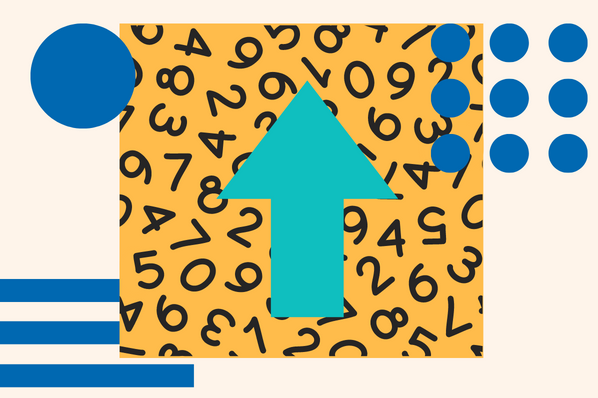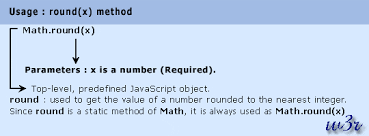# The Complete Guide for Rounding Numbers in Javascript

Published:

JavaScript offers various methods to round numbers, which is a frequent task in programming. Rounding is beneficial while handling math calculations, financial information, and presenting data to users. Rounding a number to a specific precision or an integer is often necessary.The focus of this blog post is to discuss the various rounding methods that can be used in JavaScript. We’ll provide practical examples to aid understanding and discuss best practices when rounding numbers to achieve consistency and accuracy in code. Whether you’re a new or experienced coder, this guide will help you build a strong foundation for rounding numbers in JavaScript.## What is JavaScript round?Image Source

The JavaScript round method can be used to round a number to its nearest integer. This method considers the value of the fractional part of the number to determine whether to round up or down. This is a built-in method available in JavaScript.

The syntax for the round method is as follows:

 Math.round(number)

Where “number” is the number to be rounded. The Math object is a built-in object in JavaScript that provides mathematical constants and functions, including the round method.

The round method is useful for financial data and number display, as it ensures clear formatting. Nonetheless, it only rounds to the nearest integer and lacks precision control. More advanced techniques such as ceil, floor, and trunc should be used for precision in rounding.

## How to Use Javascript Round

These are the steps to use the round method in JavaScript:

### 1. Identification

To round a number, first identify the variable, constant, or function output you wish to round.

### 2. Enter the code.

To round a number to the nearest integer, you can use Math.round() method and pass in the number as an argument. The following code is an example of rounding the number 4.6:

 const num = 4.6;const rounded = Math.round(num);console.log(rounded); // Output: 5

### 3. Rounding Up

The round method in this program returns the integer value of the rounded number. For instance, in the above example, when the number 4.6 was rounded, the returned value was 5.

### 4. Negative Numbers

Negative numbers can also be rounded using the round method. To illustrate, if you want to round the number -4.6 to the nearest integer, you can use the following code:

 const num = -4.6;const rounded = Math.round(num);console.log(rounded); // Output: -5

In this case, the round method rounds the number down to the nearest integer, which is -5.

You can now use the rounded value as per your preference, whether it is to showcase it on a webpage or include it in a calculation.

## Rounding Methods in JavaScript

JavaScript offers various other methods for rounding numbers apart from the round() method. These methods are also available in the language, and they can be used accordingly.

### 1. Math.ceil():

The following code demonstrates how to round a number up to the nearest integer, no matter what it is. For instance, when you execute this code, the number 4.2 will be rounded up to 5:

 const num = 4.2;const rounded = Math.ceil(num);console.log(rounded); // Output: 5

### 2. Math.floor():

The following code demonstrates how to use this method to round down a number to the nearest integer, no matter what it is. For instance, if you use this method with the number 4.9, the result will be 4:

 const num = 4.9;const rounded = Math.floor(num);console.log(rounded); // Output: 4

### 3. Math.trunc():

This method truncates the decimal part of a number towards zero, meaning it removes the decimal part without rounding the number. As an example, the code provided removes the decimal part of 4.5 and outputs 4 as the result:

 const num = 4.5;const truncated = Math.trunc(num);console.log(truncated); // Output: 4

Note that the trunc method solely eliminates the decimal portion of a number and does not perform any rounding.

### 4. toFixed():

This method formats the given number as a string with a defined number of decimal places. To illustrate, if we round the number 4.55 to two decimal places, the method would output the string “4.56.”

 const num = 4.55;const rounded = num.toFixed(2);console.log(rounded); // Output: “4.56”

This method can be useful when you need to display a number with a specific number of decimal places, such as when working with financial data.

## Getting Started

JavaScript provides different methods for rounding numbers depending on the required level of precision. The round method is the easiest and most widely used for rounding to the nearest integer. There are other methods like ceil, floor, trunc, and toFixed that can be used when more precision is needed.

Understanding the various rounding methods available in JavaScript is crucial for every developer, as rounding numbers is a fundamental operation in programming.Topics: Javascript

## Related Articles

We're committed to your privacy. HubSpot uses the information you provide to us to contact you about our relevant content, products, and services. You may unsubscribe from these communications at any time. For more information, check out our Privacy Policy.